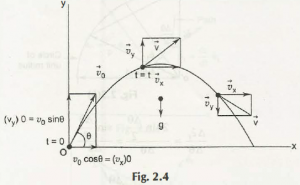When stating about projectile motion, in simple words, it is stated as a two dimensional motion. Any particle body when thrown near the surface of theearth, it moves in a certain motion along its curved path, that too under the action of earth’s gravity. That motion is called projectile motion.

After analyzing from a graph, you will find that there isn’t any presence of x component of gravity.

From that analysis, you can see,

ax= 0, and

ay = -gLet initial velocity be, (vx )0

When initial velocity remains the same throughout the projectile motion, another equation comes to the forefront.

V x = (vx)0

vy = (vy) 0 –gt

x = x0 +(vx)0 t

y = y0 +(vy)0 t -gt2

v2y = (v y)20 – 2g (y – y0)

After calculation, if you do not find x0 and y0in the initial phase, which you can find as x0 = 0 and y0 = 0, then you need to start the same calculation from the beginning.

Links of Previous Main Topic:-

Links of Next Mechanical Engineering Topics:-

### Submit Your Assignment### Customer Reviews

My Homework Help
Rated 5.0 out of 5 based on 510 customer reviews at